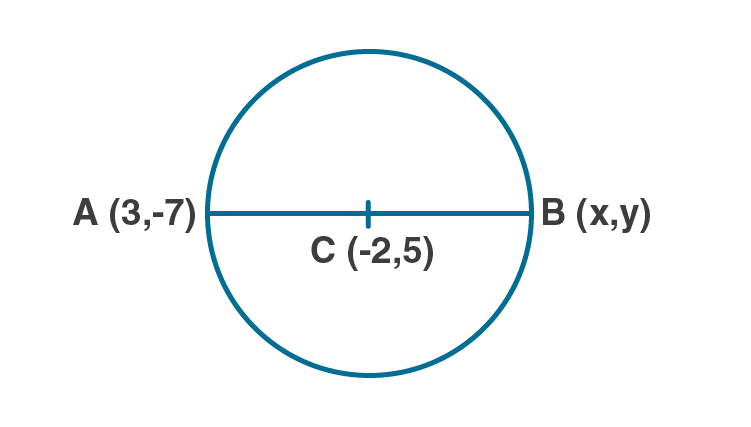Newbie

# AB is a diameter of a circle with centre C ( – 2, 5). If point A is (3, – 7). Find: (i) the length of radius AC. (ii) the coordinates of B.

• 0

An Important Question of M.L Aggarwal book of class 10 Based on Section Formula Chapter for ICSE BOARD.
In this question diameter of circle is given with its centre. You have to find the length of radius and coordinates of a point asked in question.
This is the Question Number 12, Exercise 11 of M.L Aggarwal.

Share

1.(i) Length of radius AC = d(A,C)

Co-ordinates of A = (3,-7)

Co-ordinates of C = (-2,5)

Here x1 = 3, y1 = -7, x2 = -2, y2 = 5

By distance formula, d(A,C) = √[(x2-x1)2+(y2-y1)2]

= √[(-2-3)2+(5-(-7))2]

= √[(-5)2+(12)2]

= √[25+144]

= √169

= 13

Hence the radius is 13 units.

(ii)Given AB is the diameter and C is the centre of the circle.

By midpoint formula, -2 = (x+3)/2

-4 = x+3

x = -4-3 = -7

By midpoint formula, 5 = (-7+y)/2

10 = -7+y

y = 10+7 = 17

Hence the co-ordinates of B are (-7,17).

• 2